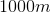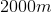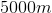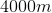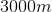# MCAT Physical : Other Flow Principles

## Example Questions

### Example Question #1 : Other Flow Principles

A barrel of height 10m is filled with water to a height of 5m. If a hole is punctured 2m above the bottom of the barrel, what is the velocity of the water leaving the barrel?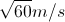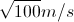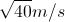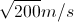Explanation:

To answer this question, we can use Toricelli’s law.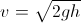In this case, h is the height of water above the hole. Since the barrel is filled to a height of 5m, and the hole is punctured 2m from the bottom of the barrel, the height of water is 3m.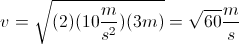### Example Question #2 : Other Flow Principles

What causes the lift experienced by the wing of an airplane in level flight?

The difference in air density on the top and bottom surfaces of the wing

The "attack angle" of the wing on the air stream

The difference in absolute humidity between the upper and lower surfaces of the wing

The difference in velocity of airflow on the top and bottom surfaces of the wing

The ability to create turbulence on the upper surface of the wing

The difference in velocity of airflow on the top and bottom surfaces of the wing

Explanation:

Air density is considered to be uniform in Bernoulli's equation, which defines the air pressure on the upper and lower surfaces of an aircraft wing. Likewise, air humidity at any one place in the atmosphere is uniform over the space occupied by the wing. Central to the idea of producing lift is the ability of the wing to avoid turbulence, one reason for de-icing wings before winter takeoffs.

Air is considered to move in bulk during flight. This means that a volume of air that has to travel a longer distance than its twin volume (because the airplane wing split them apart) must speed up to arrive at the same place (the trailing edge of the wing) at the same time as its twin. Velocity is squared in Bernoulli's equation, meaning that small differences in flow velocity between the upper and lower wing surfaces translate to large pressure differences.

Attack angle is an important lift factor, but not in level flight.

### Example Question #21 : Flow

Diffusion can be defined as the net transfer of molecules down a gradient of differing concentrations. This is a passive and spontaneous process and relies on the random movement of molecules and Brownian motion. Diffusion is an important biological process, especially in the respiratory system where oxygen diffuses from alveoli, the basic unit of lung mechanics, to red blood cells in the capillaries.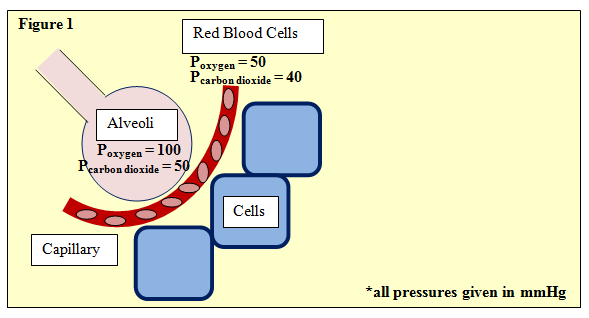Figure 1 depicts this process, showing an alveoli separated from neighboring cells by a capillary with red blood cells. The partial pressures of oxygen and carbon dioxide are given. One such equation used in determining gas exchange is Fick's law, given by:

ΔV = (Area/Thickness) · Dgas · (P1 – P2)

Where ΔV is flow rate and area and thickness refer to the permeable membrane through which the gas passes, in this case, the wall of the avlveoli. P1 and P2 refer to the partial pressures upstream and downstream, respectively. Further, Dgas­, the diffusion constant of the gas, is defined as:

Dgas = Solubility / (Molecular Weight)^(1/2)

In higher altitudes, a decrease in which factors of Fick's law can change in order to achieve the same flow rate at lower altitudes.

Thickness

Area

P1

Solubility

Thickness

Explanation:

It should be known that in higher altitudes, the partial pressure of oxygen falls. That is, the partial pressure in the alveoli will fall. Thus, flow rate will decrease, and we will need changes to increase flow rate.

By looking at Fick's equation, we can see that a decrease in thickness can help restore flow rate. Biologically speaking, this is less likely to happen, and more correctly, hemoglobin concentration and binding affinity to oxygen increases; however, this is extraneous information not needed for the MCAT.

### Example Question #4 : Other Flow Principles

Diffusion can be defined as the net transfer of molecules down a gradient of differing concentrations. This is a passive and spontaneous process and relies on the random movement of molecules and Brownian motion. Diffusion is an important biological process, especially in the respiratory system where oxygen diffuses from alveoli, the basic unit of lung mechanics, to red blood cells in the capillaries.Figure 1 depicts this process, showing an alveoli separated from neighboring cells by a capillary with red blood cells. The partial pressures of oxygen and carbon dioxide are given. One such equation used in determining gas exchange is Fick's law, given by:

ΔV = (Area/Thickness) · Dgas · (P1 – P2)

Where ΔV is flow rate and area and thickness refer to the permeable membrane through which the gas passes, in this case, the wall of the avlveoli. P1 and P2 refer to the partial pressures upstream and downstream, respectively. Further, Dgas­, the diffusion constant of the gas, is defined as:

Dgas = Solubility / (Molecular Weight)^(1/2)

Conceptually, if alveoli are considered to be perfectly spherical and assuming that its entire surface exchanges gas, which new relationship, introducing the variable, r, the radius of an alveoli, correctly describes Fick's Equation, assuming partial pressures remain constant?

ΔV = (1/2 · r1/2)/Thickness · Dgas · (P1 – P2)

ΔV = 4πr2/Thickness · Dgas · (P1 – P2)

ΔV = r3/Thickness · Dgas · (P1 – P2)

ΔV = πr2/Thickness · Dgas · (P1 – P2)

ΔV = 4πr2/Thickness · Dgas · (P1 – P2)

Explanation:

The quickest approach to this equation is to see what variable in Fick's law might be affected by a change in the radius of an alveoli. Pressure is constant and can be ruled out. The diffusion constant would not be effected by radius (hence it being a constant).

The question stem mentions nothing about a change in thickness, therefore, we are left with area.

The surface area of a sphere is measured by 4πr2 and can be substituted. If you had difficulty remembering how to measure surface area, remember that the area of a circle is πr2 andlogically it follows that the surface area of an entire sphere must be greater due to it being three dimensional.

### Example Question #5 : Other Flow Principles

Diffusion can be defined as the net transfer of molecules down a gradient created by differing concentrations of the molecule in different locations. This is a passive, spontaneous process and relies on the random movement of molecules and Brownian motion. Diffusion is an important biological process, especially in the respiratory system where oxygen diffuses from alveoli, the basic units of lung mechanics, to red blood cells in the capillaries.Figure 1 depicts this process, showing an alveolus separated from neighboring cells by a capillary with red blood cells. The partial pressures of oxygen and carbon dioxide are given. One equation used in determining gas exchange is Fick's law, given by: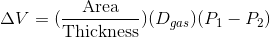In this equation,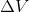is the flow rate. Area and thickness refer to the permeable membrane through which the gas passesin this case, the wall of the alveolus.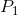and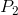refer to the partial pressures upstream and downstream, respectively.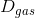, the diffusion constant of the gas, is defined as: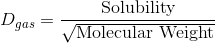At high altitudes, the partial pressure of oxygen quickly drops while that of carbon dioxide decreases by much less. Given the following table, at what elevation is the pressure gradient of oxygen equal to half that of carbon dioxide, assuming constant capillary partial pressures of oxygen and carbon dioxide of 50mmHg and 40mmHg, respectively?

 Elevation (m) PO2 (mmHg) P­­CO2 (mmHg) 0 100 50 1000 80 40 2000 60 30 3000 40 20 4000 20 10 5000 10 0